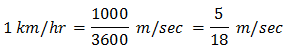1. Worksheets>
2. Math>
3. Solve>
4. Speed>
5. Conversion 1

# How to convert km/hr into m/sec

1 km = 1000 m; 1 hr = 3600 sec.To convert km/hr into m/sec, multiply the number by 5 and then divide it by 18.

Need detail explanation? Go to bottom

Solved Example 1:

Convert 54 km/hr into m/sec.

Solution:

54 km/hr

Step 1:

Multiply 54 by 5

We have 54 * 5 = 270

Step 2:

Divide 270 by 18

270/18 = 15

54 km/hr = 15 m/sec

Try yourself: Practice Sheet 1

Solved Example 2:

Convert 120 km/hr into m/sec. Round the answer to 2 decimal places.

Solution:

120 km/hr

Step 1:

Multiply 120 by 5

We have 120 * 5 = 600

Step 2:

Divide 600 by 18

600/18 = 33.3333... = 33.33

120 km/hr = 33.33 m/sec

Try yourself: Practice Sheet 2

Better Understanding:

Imagine the situation of buying apples.

Suppose an apple cost 25 cents, what is the cost of 2 apples? Yes, it is 2 * 25 = 50 cents. Cost of 3 apples = 3 * 25 = 75 cents.

Let us work out the problem in example 1 as it explained here.